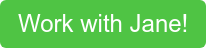One topic that seemed a bit mysterious and magic to me when I first learned calculus was implicit differentiation. In this post, we’ll start by reviewing some examples of implicit differentiation and then discuss why implicit differentiation works.

An example

In an implicit differentiation problem, you’re generally given an equation involving x and y such asThen, you’re asked to find the derivative of y with respect to x. One way to do this is to solve for y with respect to x and then take the derivative normally. Another way is via implicit differentiation by differentiating both sides with respect to x.

Doing so, we get:This is the prime time to use implicit differentiation because it allows us to go straight to differentiating both sides rather than worry about isolating y, which could be difficult.

What is the intuition behind implicit differentiation?

Let’s explore the idea behind implicit differentiation via an example. Suppose that we have the equation of a circle of radius 5,In other words, we can take the derivatives of both sides with respect to x and these derivatives must always be the same.So if we wanted the slope of the tangent line of the circle of radius 5 at the point (3,4) for example, we could use the derivative that we just found to get that the slope of the tangent line there is -4/3.

In conclusion

Implicit differentiation is a super important tool when finding derivatives when x and y are related not by y=f(x) but by a more complicated equation. By remembering that two sides of an equation are always equal and therefore their derivatives with respect to x must be equation, and remembering that y depends on x, we can carefully take derivatives of both sides with respect to x, rearrange, and then solve forThis allows us to find derivatives even in cases when it’s hard to write y as a function of x.

Mathematics – from high school math to graduate school math – is one of our most frequently requested subjects. Teaching math is notoriously difficult and we maintain a staff of mathematicians who are committed to the art of teaching. There is no course or standardized test that we do not have extensive experience teaching. We work with students who loathe math and students who love it, students who haven’t done math in a decade and students who work on mathematical problems every day. Many of our students work with tutors to address courses or exams – such as Geometry, Linear Algebra, Differential Equations – but we also work with students looking to explore more advanced or unconventional topics (like the mathematics of poker, or algrabraic topology, for example).Read some more of Jane's blog posts below!

Tags: math